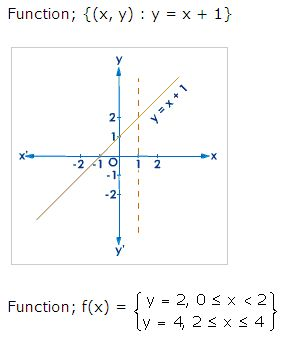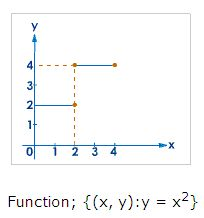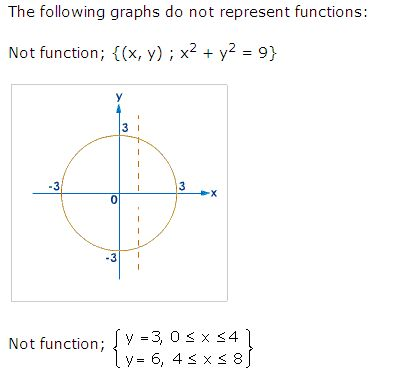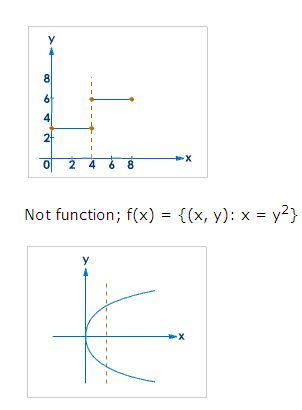Name: ___________________Date:___________________

 Email us to get an instant 20% discount on highly effective K-12 Math & English kwizNET Programs!

High School Mathematics - 24.1 Test of Functions: Vertical Line Test

 The vertical line test One way to tell if a graph is a function is the vertical line test, which states that if it is possible for a vertical line to meet a graph more than once, the graph is not a function. Method to test a function: Draw a line parallel to the y-axis. If this line cuts the graph at one distinct point, then it is a function otherwise it is not a function.Name: ___________________Date:___________________

High School Mathematics - 24.1 Test of Functions: Vertical Line Test

 Q 1: If every vertical line intersect a graph in no more than one point, then the graph is the graph of a function.truefalse Q 2: You can use one of the following to perform a vertical line test.protractorrulercompass Q 3: A wave graph is a function.truefalse Q 4: Is circle a function?yesno Q 5: A vertical line with x-intercept a has an equation of the form x = a. Is this line a function.noyes Q 6: The vertical line test is a check to see if a graph of a relation is a ________.Answer: Question 7: This question is available to subscribers only! Question 8: This question is available to subscribers only!